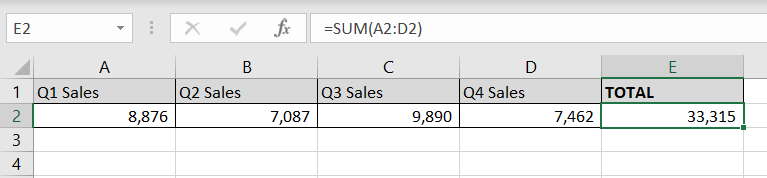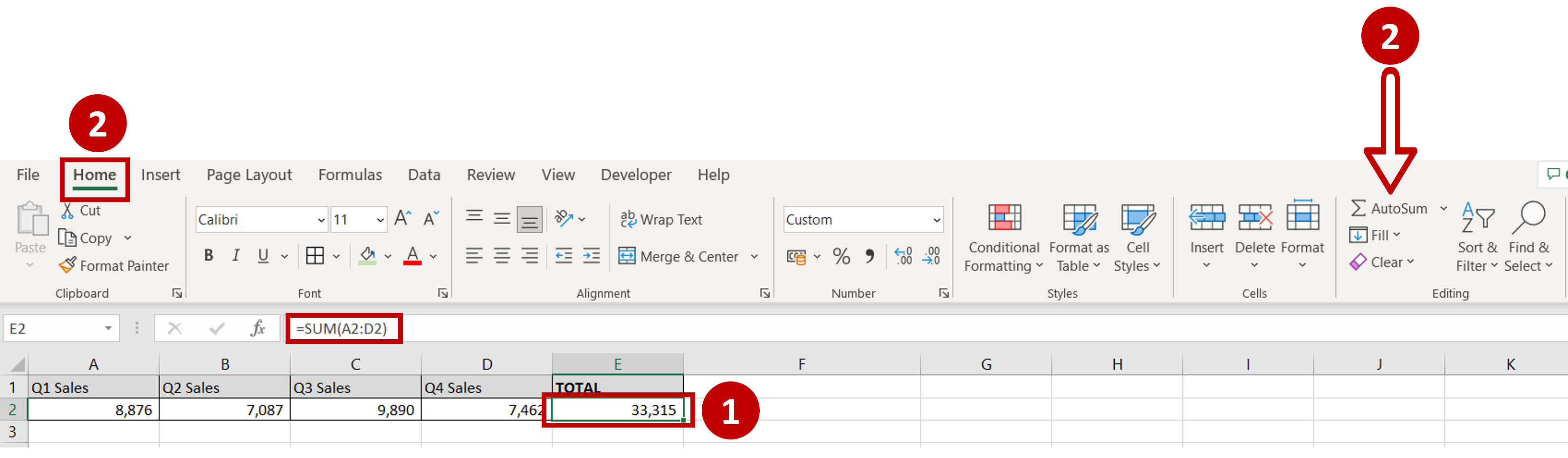# How to add a range of cells in Excel

You can watch a video tutorial here.Excel is widely used for calculations due to the several arithmetic operators and functions that it has. Instead of typing in numbers for calculations, you can use the cell references i.e. the address (Column header & Row number) of the cell in the calculation. When adding a range of cells in Excel, you can do so using the cell references of the start and end of the range.

### Step 1 – Use AutoSum